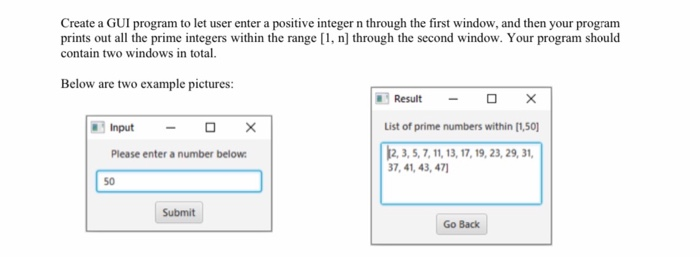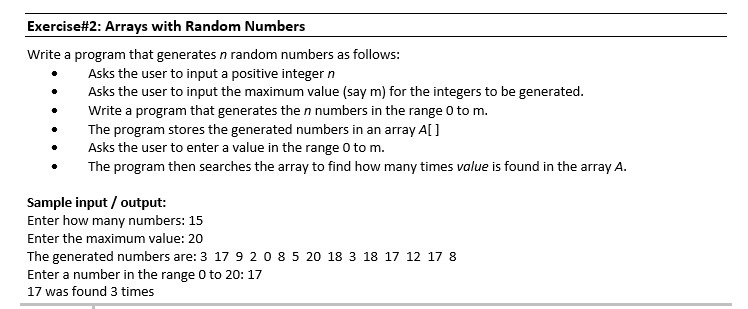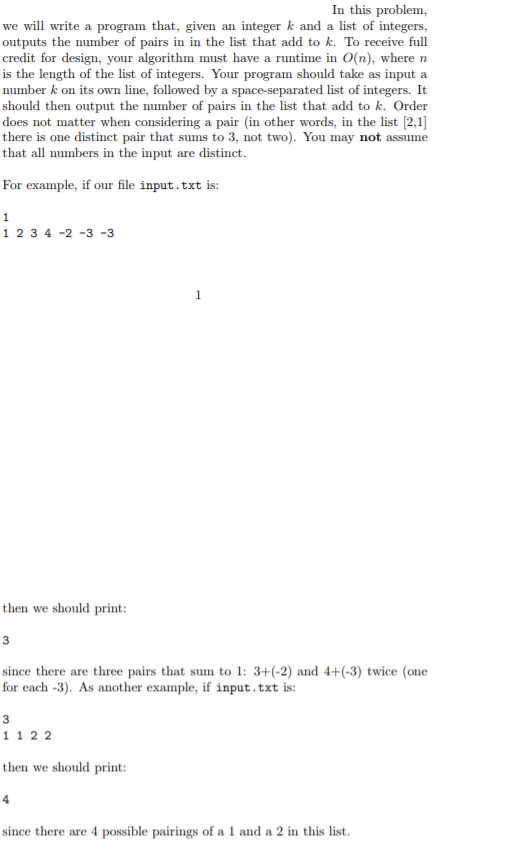Question

# Finding number pairs that add to n without using arrays or hashing

## Problem

Given a number n between 1 and 12 (inclusive), your job is to find all the pairs of numbers that produce n when summed. For instance, given the number 5, two possible pairs of numbers are`1, 4` and `2, 3`. Each number in the pair must be unique, meaning that `3, 3` is not a valid pair to sum to 6.
You will be writing a method to accomplish this goal.

## sumOfPairs()

Your method will take as a parameter the integer n to find sums of.
Your method should return a String containing all of the pairs of numbers that sum to n. If there are no such pairs, you should return the String “NONE”.

## Main Method

You should also fill in the `main` method to test the `sumOfPairs()` method.
Your `main` method should get user input, then call the method with the value retrieved. The user shouldn’t be allowed to enter a number outside of the available range (1 - 12 inclusive). Your program should continue asking for input until the user enters 0.

## Sample Run

```Enter a number between 1 and 12 inclusive (0 to quit): 2
Pairs for 2: NONE
Enter a number between 1 and 12 inclusive (0 to quit): 3
Pairs for 3: 1 2
Enter a number between 1 and 12 inclusive (0 to quit): 5
Pairs for 5: 1 4, 2 3
Enter a number between 1 and 12 inclusive (0 to quit): 15
Out of range, try again!
Enter a number between 1 and 12 inclusive (0 to quit): 0```

good luck body

#### Earn Coins

Coins can be redeemed for fabulous gifts.

Similar Homework Help Questions
• ### Sum of Pairs

Sum of PairsProblemGiven a number n between 1 and 12 (inclusive), your job is to find all the pairs of numbers that produce n when summed. For instance, given the number 5, two possible pairs of numbers are1, 4 and 2, 3. Each number in the pair must be unique, meaning that 3, 3 is not a valid pair to sum to 6.You will be writing a method to accomplish this goal.sumOfPairs()Your method will take as a parameter the integer ...

• ### Write a program using M.I.P.S that asks user “how many positive number that is devisable by...

Write a program using M.I.P.S that asks user “how many positive number that is devisable by 6 you want to add?” .Then your loopcounter would be the user input. If the user enters a positive number between 1 and 100 that is devisable by 6, you increment your loop counter and add it to the sum.. You need to decide if the positive number entered by the user is divisible by 6 or not. Your program should print an error...

• ### in java the program must contain the main method and example( the number 50 should be...in java the program must contain the main method and example( the number 50 should be the input) must be included in the main method to check the correctness of your programs. Create a GUI program to let user enter a positive integer n through the first window, and then your program prints out all the prime integers within the range [1, n) through the second window. Your program should contain two windows in total. Below are two example pictures:...

• ### use c++ language, keep it simple i am using code block Exercise#2: Arrays with Random Numbers...use c++ language, keep it simple i am using code block Exercise#2: Arrays with Random Numbers Write a program that generates n random numbers as follows: Asks the user to input a positive integer n Asks the user to input the maximum value (say m) for the integers to be generated. Write a program that generates the n numbers in the range 0 to m. The program stores the generated numbers in an array A[ Asks the user to enter...

• ### Loops | Methods | Arrays(programming language java) in software (ATOM) Write a toolkit that includes some...

Loops | Methods | Arrays(programming language java) in software (ATOM) Write a toolkit that includes some common functions a user might want or need An encryption/ decryption method. Both methods should take in a String (the String that needs to be changed), and an encryption value. The methods need to change the String by the value Write a method that takes an integer array as an argument. The method should return the sum of all of the elements Write a...

• ### Import java.util.*; public class PairFinder { public static void main(String[] args) { Scanner sc...import java.util.*; public class PairFinder { public static void main(String[] args) { Scanner sc = new Scanner(System.in);    // Read in the value of k int k = Integer.parseInt(sc.nextLine());    // Read in the list of numbers int[] numbers; String input = sc.nextLine(); if (input.equals("")) { numbers = new int; } else { String[] numberStrings = input.split(" "); numbers = new int[numberStrings.length]; for (int i = 0; i < numberStrings.length; i++) { numbers[i] = Integer.parseInt(numberStrings[i]); } }    System.out.println(findPairs(numbers, k)); }    //method that...

• ### This C++ Programs should be written in Visual studio 2017 PROGRAM 1: Comparing Using Arrays to...

This C++ Programs should be written in Visual studio 2017 PROGRAM 1: Comparing Using Arrays to Using Individual Variables One of the main advantages of using arrays instead of individual variables to store values is that the program code becomes much smaller. Write two versions of a program, one using arrays to hold the input values, and one using individual variables to hold the input values. The programs should ask the user to enter 10 integer values, and then it...

Please please help with the code! Apperciate all the effort --create using html5, css3, and javascript. --The interface permits the user to compute the number of primes in ranges of integer values. --The interface should present the user with 4 pairs of "number" boxes in which 4 pairs of integer values can be entered. Each pair of values should be used by a thread to compute the number of prime numbers in that range, inclusive. -- If any box is...

• ### In this lab, you complete a partially written Java program that includes two methods that require...

In this lab, you complete a partially written Java program that includes two methods that require a single parameter. The program continuously prompts the user for an integer until the user enters 0. The program then passes the value to a method that computes the sum of all the whole numbers from 1 up to and including the entered number. Next, the program passes the value to another method that computes the product of all the whole numbers up to...

• ### In this lab, you complete a partially written Java program that includes two methods that require...

In this lab, you complete a partially written Java program that includes two methods that require a single parameter. The program continuously prompts the user for an integer until the user enters 0. The program then passes the value to a method that computes the sum of all the whole numbers from 1 up to and including the entered number. Next, the program passes the value to another method that computes the product of all the whole numbers up to...